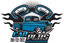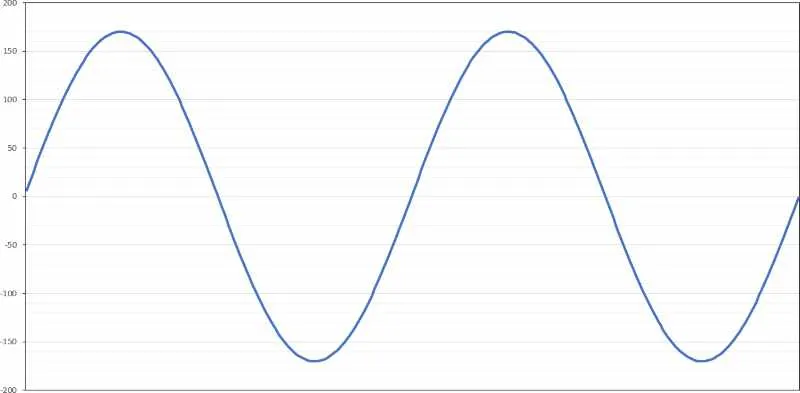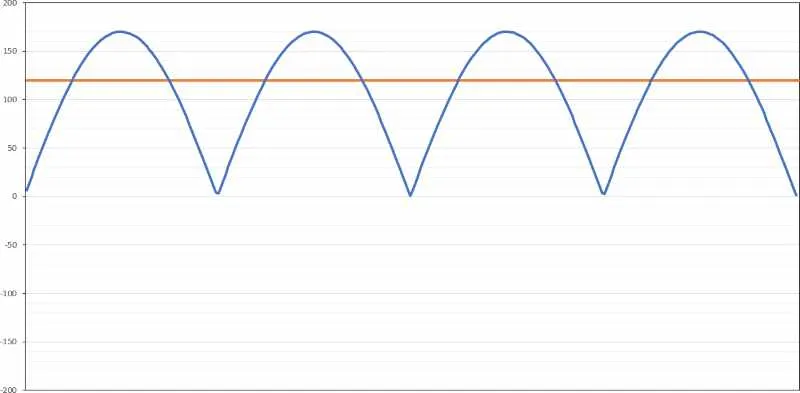Search
•Car Plus

# What RMS and Peak Power Ratings Really MeanAnother scrolling session on Reddit is complete, and it gave me an idea for an article that might help explain a common question. When people shop for amplifiers, they often see the terms “RMS” and “peak” regarding power ratings. What do these terms mean, and are they an accurate way to quantify how car audio products will perform? Let’s find out!

## Power Measurements in Direct Current Circuits

In an electrical circuit that uses a direct current power source, calculating the power consumed by the load is simple. If you multiply the voltage across the load by the current through the load, you’ll get the number of watts dissipated in the device. For example, if we have a lightbulb connected to a 12-volt battery and 5 amps of current flow through the wires going to and from the bulb, then that bulb dissipates 60 watts of energy.

It’s also worth noting that this light bulb might not produce 60 watts of light. This is because some of the energy going into the bulb is converted to heat. Incandescent light bulbs are notoriously inefficient. They convert 95% of the energy from the power source into heat, and only 5% into light. For our 60-watt bulb, that’s only 3 watts of light energy. So we would describe this bulb as being 5% efficient in terms of a light source but 95% efficient as a heat source.

## Power Measurements in Alternating Current Circuits

When calculating power in circuits that use alternating current, the math becomes a little more complicated. Unlike a DC circuit, in an AC circuit the voltage across the load varies with time. For example, in our homes, the power receptacles on the wall provide about 120 volts of electricity. Did you know that this is the RMS value and not the peak?

If you were to look on an oscilloscope at the sinusoidal AC voltage waveform coming into your home, you’d see that the voltage swings from plus 170 to minus 170 volts. Work is done by whatever you plug into the wall outlet, whether the voltage is positive or negative at any moment in time. In the case of most consumer electronics, the AC voltage is rectified to a DC voltage, but that’s a whole other crazy topic.

If we look at the amount of power available from an AC signal to do work, we need to make a few calculations. The graph below shows the 340-volt peak-to-peak waveform.The first step in determining how much work this AC voltage waveform can do is to cut the voltage in half. Think of it this way: The voltage pushes or pulls current, and our load doesn’t care which way the current flows. The ability to do work or flow current is the distance the voltage waveform is away from the ground voltage level (which we hope is 0V). So, the maximum power in the circuit is the difference between the ground and the positive or negative voltage peak.## What RMS and Peak Power Ratings Really Mean

January 3, 2022 By Dave MacKinnon

Another scrolling session on Reddit is complete, and it gave me an idea for an article that might help explain a common question. When people shop for amplifiers, they often see the terms “RMS” and “peak” regarding power ratings. What do these terms mean, and are they an accurate way to quantify how car audio products will perform? Let’s find out! Power Measurements in Direct Current Circuits In an electrical circuit that uses a direct current power source, calculating the power consumed by the load is simple. If you multiply the voltage across the load by the current through the load, you’ll get the number of watts dissipated in the device. For example, if we have a lightbulb connected to a 12-volt battery and 5 amps of current flow through the wires going to and from the bulb, then that bulb dissipates 60 watts of energy. It’s also worth noting that this light bulb might not produce 60 watts of light. This is because some of the energy going into the bulb is converted to heat. Incandescent light bulbs are notoriously inefficient. They convert 95% of the energy from the power source into heat, and only 5% into light. For our 60-watt bulb, that’s only 3 watts of light energy. So we would describe this bulb as being 5% efficient in terms of a light source but 95% efficient as a heat source. Power Measurements in Alternating Current Circuits When calculating power in circuits that use alternating current, the math becomes a little more complicated. Unlike a DC circuit, in an AC circuit the voltage across the load varies with time. For example, in our homes, the power receptacles on the wall provide about 120 volts of electricity. Did you know that this is the RMS value and not the peak? If you were to look on an oscilloscope at the sinusoidal AC voltage waveform coming into your home, you’d see that the voltage swings from plus 170 to minus 170 volts. Work is done by whatever you plug into the wall outlet, whether the voltage is positive or negative at any moment in time. In the case of most consumer electronics, the AC voltage is rectified to a DC voltage, but that’s a whole other crazy topic. If we look at the amount of power available from an AC signal to do work, we need to make a few calculations. The graph below shows the 340-volt peak-to-peak waveform. The first step in determining how much work this AC voltage waveform can do is to cut the voltage in half. Think of it this way: The voltage pushes or pulls current, and our load doesn’t care which way the current flows. The ability to do work or flow current is the distance the voltage waveform is away from the ground voltage level (which we hope is 0V). So, the maximum power in the circuit is the difference between the ground and the positive or negative voltage peak. The graph above shows a waveform that can do the same amount of work as our sine wave. The peak voltage is 170 volts relative to the ground. Since our light bulb doesn’t care how the current flows, it does the same amount of work. The last thing we have to deal with to understand the energy provided by an AC circuit is to average out the peaks and dips. So we calculate the waveform’s root mean square value, to be more specific. The RMS voltage is a DC-equivalent voltage capable of doing the same amount of work as an AC voltage. The calculation for a pure sine wave is V-peak ÷ √2. To make the math more manageable, you can replace the √2 with 1.414; that’s close enough. If we apply this to our 170 volt-peak waveform, we get 120.23 volts – call it 120 volts. The orange line on the graph below shows the RMS value of this 170-volt peak waveform.We’ve added some information to the last chart to help you understand and visualize how this RMS voltage calculation works. The portion of the AC voltage waveform above the RMS value is filled in with green. The area where the waveform is below the RMS value is yellow. Think of it this way: The green area fills in the yellow area, and we are left with an equivalent DC voltage level we can use to calculate the work that the voltage can do. This description isn’t exactly how it works, but it explains the concept as a generalization. Another caveat is that this calculation only works for purely resistive loads. Items like speakers are reactive, so phase differences between current and voltage need to be considered. But, once again, that’s fodder for a different article.## Alternating Current and Power Calculations

When an engineer or technician tests an amplifier, they use sine waves. The test equipment (be it an audio analyzer or a true RMS multimeter) automatically takes care of this V-peak ÷ √2 calculation when displaying information. For example, if we play a sine wave at 60 Hz with a peak-to-peak measurement of 340 volts or a peak measurement of 170 volts, the meter will show 120 volts. To calculate power, we take that voltage number, square it, then divide by the load resistance. If you connect a 4-ohm speaker to the wall outlet, you’ll get 3,600 watts RMS. Unfortunately, the circuit will need to provide 30 amps of current, so the fuse or breaker in the electrical panel will pop right away. Using an 8-ohm speaker, you get 1,800 watts, and the load draws 15 amps. Anyways, please don’t connect your subwoofers to the wall.

Just when you thought everything was going swimmingly, we’ll throw a wrench into the mix. Let’s say we have an AC waveform that’s clipped and looks like a square wave. See the graph below.Now, if we flip the negative voltage over to a positive and fill in any gaps, we get 170 volts because there are no gaps.

If our amplifier is clipping like crazy and producing an audio signal full of harmonics that looks like a square wave, the amount of power the amplifier is producing has doubled. The RMS voltage calculation doesn’t need the √2 multiplier because there are no valleys in the waveform. If we square this voltage (170) and divide it by the 4-ohm load, we get 7,225 watts – pretty close to double 3,600 watts, right? If you’ve ever wondered how a 300-watt amp can damage a 400-watt subwoofer, this is why. When pushed beyond its ability to produce a clean waveform, that amp can make around 600 watts. That’s the theory; at least, it’s likely closer to 500 watts in practice, but it’s still more than 400 watts.

## What Do Amplifier Manufacturers Mean by RMS and Peak?

When a reputable car audio amplifier manufacturer specifies as power rating as RMS, they’re attempting to describe how much power the amp can make before the signal starts to distort significantly. Let’s say we have an amp rated to produce 600 watts into a 2-ohm load. The audio waveform coming out of the amp at full power would be 34.6 volts RMS, or 48.98 volts peak, or 97.96 volts peak-to-peak. All three numbers describe the same value.The graph above shows our 97.96-volt peak-to-peak waveform with the 48.98 peak voltage and 34.6 volt RMS level in orange.

Of course, the amplifier can try to make more power if we increase the sensitivity control or feed more signal into it. The peak voltage won’t increase, but the RMS voltage will. It takes some fancy math to calculate the RMS signal level when the waveform isn’t a sine or square wave. That’s beyond the scope of this article. The graph below shows an approximation of the increase in RMS power level if we push that amplifier into clipping.If a car audio manufacturer wants to accurately describe how much power an amplifier makes, they should follow the guidelines associated with that specification. For our industry, we have the ANSI/CTA-2006-C standard. It specifies that the power rating measurements include no more than 1% distortion and noise, that the amplifier cannot be powered with more than 14.4 volts and that the measurement bandwidth is specified. You’ll often see a rating written as 600 Watts RMS @ <1% THD+N, 20 Hz to 20 kHz at 14.4 Volts. If any of those quantifiers is excluded, you should question the validity of the spec. As a note for subwoofer amplifiers, the frequency response spec might be something like 20 to 250 Hz; that’s fine.

So, what does a peak rating refer to if the above CTA-2006-C spec is implied by stating RMS? Well, in short, it’s a useless number that describes how much power the amp can produce when the audio signal is distorted with harmonic content, as we see in the above graph. Nobody should want to play their music with the amplifier running in this range since it will sound terrible. More often than not, it’s a vague calculation based on how much power the amplifier consumes and ignores the circuitry efficiency, or it’s an outright fabrication that remains irrelevant to any discussion of useful power available to move a speaker.

## Shop for Car Audio Amplifiers with Proper Specifications

Though this got a little mathematical, the concept of evaluating the ability of an AC voltage to do work is relatively simple. The takeaway is that you can ignore peak power ratings and only consider those labeled with RMS when the appropriate qualifications are stated. Your local specialty mobile enhancement retailer can steer you in the right direction to get a solution that performs the way you expect.